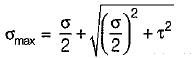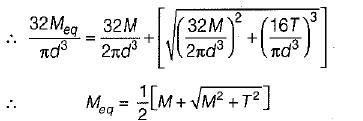Courses

# Test: Analysis of Stress & Strain - 2

## 10 Questions MCQ Test Topicwise Question Bank for Mechanical Engineering | Test: Analysis of Stress & Strain - 2

Description
This mock test of Test: Analysis of Stress & Strain - 2 for Mechanical Engineering helps you for every Mechanical Engineering entrance exam. This contains 10 Multiple Choice Questions for Mechanical Engineering Test: Analysis of Stress & Strain - 2 (mcq) to study with solutions a complete question bank. The solved questions answers in this Test: Analysis of Stress & Strain - 2 quiz give you a good mix of easy questions and tough questions. Mechanical Engineering students definitely take this Test: Analysis of Stress & Strain - 2 exercise for a better result in the exam. You can find other Test: Analysis of Stress & Strain - 2 extra questions, long questions & short questions for Mechanical Engineering on EduRev as well by searching above.
QUESTION: 1

### ‘p1’ and ‘p2’ are two equal tensile principal stresses. On the plane AB inclined at 45° to the plane of ‘p1’ as shown in the figure below,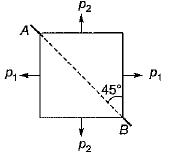Solution:

The plane AB is principal plane, and at the principal plane shear stress is zero.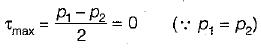QUESTION: 2

### In a Mohr’s circle, the radius of the circle is taken as

Solution: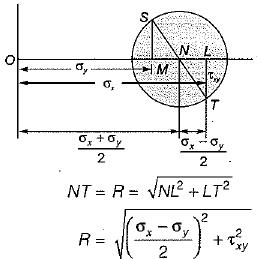QUESTION: 3

### For a general two dimensional stress system, what are the coordinates of the centre of Mohr’s circle?

Solution:

From the above figure coordinates of the centre are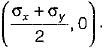QUESTION: 4

A Mohr’s circle reduces to a point when the body is subjected to

Solution:

When the normal stresses on the two mutually perpendicular planes are equal and alike then radius of Mohr’s circle will be zero.
i.e.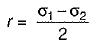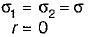Hence Mohr’s circle reduces to a point.

QUESTION: 5

If a body carries two unlike principal stresses, what is the maximum shear stress?

Solution: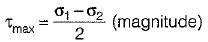The principal stress given are unlike i.e if one is tensile and other is compressive. Let σ1 be tensile and σ2 be compressive, then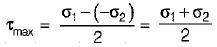QUESTION: 6

Maximum shear stress in a Mohr’s circle

Solution: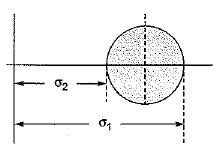Radius of the Mohr’s Circle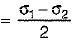Maximum shear stress,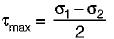∴ Radius of Mohr’s circle is equal to maximum shear stress

QUESTION: 7

In a two dimensional problem, the state of pure shear at a point is characterized by

Solution:
QUESTION: 8

In a plane stress problem there are normal tensile stresses σx and σy accompanied by shear stres τxy at a point along orthogonal Cartesian co-ordinates x and y respectively. If it is observed that the minimum principal stress on a certain plane is zero then,

Solution:

Principal stresses,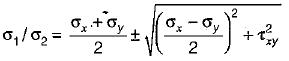Since minimum principal stress is zero, so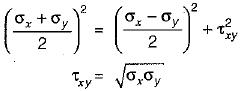QUESTION: 9

State of stress at a point in a strained body is shown in figure.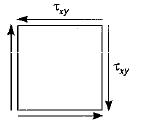Which one of the figure given below represents correctly the Mohr's circle for the state of stress?

Solution:

Mohr’s circle for pure shear stress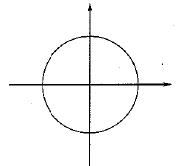Since neither tensile nor compressive stress exists, the Mohr’s diagram is simply a circle of radius τxy and centre at the intersection of the two axes. It can also be verified by transformation of plane formula.

QUESTION: 10

The equivalent bending moment under combined action of bending moment M and torque T is

Solution: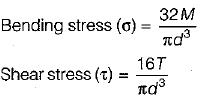Maximum principal stress,